# Partition function

Based on the finiteness position of middle zero axis = 15 we have defined our 19 vs 18 Scenario contained in three (3) groups of five (5) of integer number partitions. Each groups represents the three (3) basic arithmetic operations of Euler's identity.

Euler's identity is often cited as an example of deep mathematical beauty. Three (3) of the basic arithmetic operations occur exactly once each: addition, multiplication, and exponentiation (Wikipedia).

``````---+-----+-----
1 | 1   | 5    🡰--19--
---+-----+-----        |
2 | 6   | 8           |
---+-----+-----        |
3 | 9   | 26    --289-¤-exponentiation zone
---+-----+-----        |
4 | 27  | 28          |
---+-----+-----        |
5 | 29  | 29   ➤--18--
---+-----+-----
6 | 30  | 31   🡰--18--
---+-----+-----        |
7 | 32  | 44          |
---+-----+-----        |
8 | 45  | 46    --329-¤-multiplication zone
---+-----+-----        |
9 | 47  | 49          |
---+-----+-----        |
10 | 50  | 50   ➤--17--
---+-----+-----
11 | 51  | 53   🡰--17--
---+-----+-----        |
12 | 54  | 59          |
---+-----+-----        |
13 | 60  | 82    --168-¤-addition zone
---+-----+-----        |
14 | 83  |{102}        |
---+-----+-----        |
15 |{103}| 110  ➤--16--
---+-----+-----
``````

The five (5) of integer number partitions shows a profound connection between the most fundamental numbers in mathematics as it also links the five (5) fundamental mathematical constants:

(1) The number 1, the multiplicative identity,
(2) The number i, the imaginary unit of the complex numbers.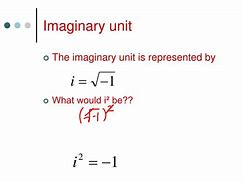(3) The number π = 3.1415…, the fundamental circle constant, and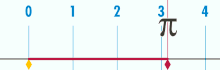(4) The number e = 2.718…, also known as Euler's number, which occurs widely in mathematical analysis.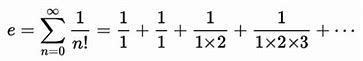(5) Furthermore, the equation is given in the form of an expression set equal to zero, the number 0, as the additive identity which is common practice in several areas of mathematics.

Euler's identity is a special case of Euler's formula eix = cos x + i sin x when evaluated for x = π, In addition, it is directly used in a proof that π is transcendental, which implies the impossibility of squaring the circle. (Wikipedia)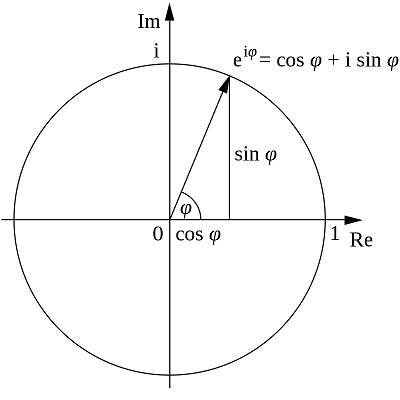It is stated by DE102011101032A9 that using Euler's identity, the MEC30 standard is more accurately than a measurement. The distribution of prime numbers is a central point of study in number theory. So let's start from there.

## Rational Objects

In number theory, the partition functionp(n) represents the number of possible partitions of a non-negative integer n. Integers can be considered either in themselves or as solutions to equations (Diophantine geometry).

The central problem is to determine when a Diophantine equation has solutions, and if it does, how many. Two examples of an elliptic curve, that is, a curve of genus 1 having at least one rational point. Either graph can be seen as a slice of a torus in four-dimensional space (Wikipedia).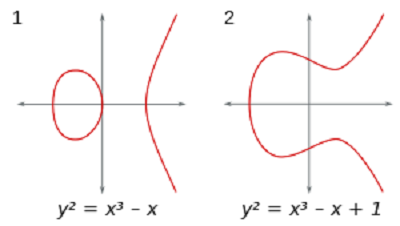One of the main reason is that one does not yet have a mathematically complete example of a quantum gauge theory in four-dimensional space-time. It is even a sign that Einstein's equations on the energy of empty space are somehow incomplete.

Throughout his life, Einstein published hundreds of books and articles. He published more than 300 scientific papers and 150 non-scientific ones. On 5 December 2014, universities and archives announced the release of Einstein's papers, comprising more than 30,000 unique documents (Wikipedia).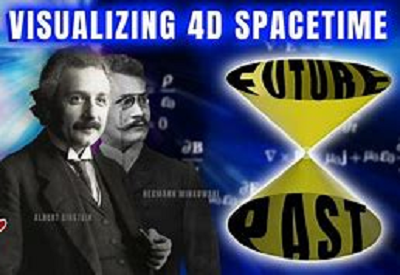Dyson introduced the concept in the context of a study of certain congruence properties of the partition function discovered by the mathematician Srinivasa Ramanujan who the one that found the interesting behaviour of the taxicab number 1729.

In number theory and combinatorics, rank of a partition of a positive integer is a certain integer associated with the partition meanwhile the crank of a partition of an integer is a certain integer associated with that partition (Wikipedia).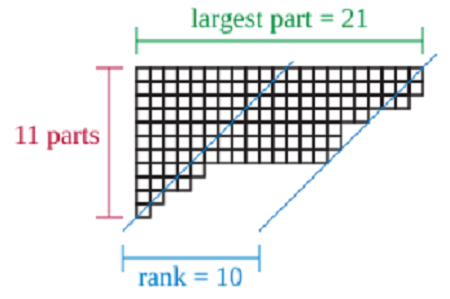Young tableaux were introduced by Alfred Young, a mathematician at Cambridge University, in 1900. They were then applied to the study of the symmetric group. Their theory was further developed by many mathematicians, including W. V. D. Hodge

### The rank of a partition

By our project, this partition stands as the prime identity. The tabulation below shows the 2nd prime identity where the 20 out of the largest part = 21 goes to rank = 10 via crank = 20-11 = 9. These 10 and 9 are associated with the 19th prime identitity.

``````           largest part = 21 → 11+13+12 = 36  →  MEC30
↓                      |
---+-----+-----+-----+-----+                   ↓
1 | 19  | 1   | 20  | 21  |-------------------|-----
---+-----+-----+-----+-----+                   ↓     |
2 | 18  | 21  | 39  | 60  |-------------------      |
---+-----+-----+-----+-----+                   |     |
3 |{63} | 40  | 103 | 143 |-------------      |     |
---+-----+-----+-----+-----+             |     |     |
4 | 37  | 104 | 141 | 245 |-------      |     |     |
---+-----+-----+-----+-----+       |     |     |     |
5 | 10* | 142 | 152 | 294 |- 11** | 13  | 12  | 12  | 18
---+-----+-----+-----+-----+       |     |     |     |
6 | 24  | 153 | 177 | 332 |-------      |     |     |
---+-----+-----+-----+-----+             |     |     |
7 | 75  | 178 | 253 | 431 |-------------      |     |
---+-----+-----+-----+-----+                   |     |
8 | 30  | 254 | 284 | 538 |-------------------      |
---+-----+-----+-----+-----+                   ↓     |
9 | 1   | 285 | 286 | 571 |-------------------|-----
===+=====+=====+=====+=====+                   ↓
45 | 277 |                      ← 11+13+12=36 ←  MEC30
---+-----+                                     |
↑
Note:
10* stands as the central rank
11** stands as the central parts
``````

By having a total of 571, the congruence properties is composed in to the last of 20/10 = 2 numbers of 285 and 286 throughout the cumulative sum of 45 objects that are forming the 10x11=110 objects of 18th prime identity.

(71+6) + (43-6) = 77 + 37 = 11x7 + 30+13-6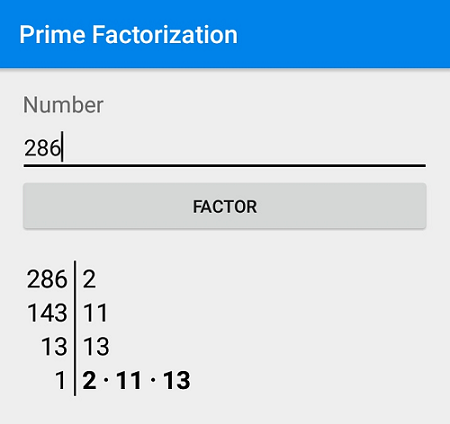This scheme of 19 vs 18 stands as our 19 vs 18 Scenario while the tensor of 110 objects are obtained by observing centralizing the first 20 to 10 objects from polarizing of 2x11x13=286 objects out of the central pair 11 and 13 within True Prime Pairs.

### The crank of a partition

In linear algebra, this tensor is known as eigenvector, a nonzero vector that changes at most by a scalar factor when that linear transformation is applied to it. The corresponding eigenvalues is the factor by which the eigenvector is scaled.

In this shear mapping the red arrow changes direction, but the blue arrow does not. The blue arrow is an eigenvector of this shear mapping because it does not change direction, and since its length is unchanged, its eigenvalue is 1 (Wikipedia).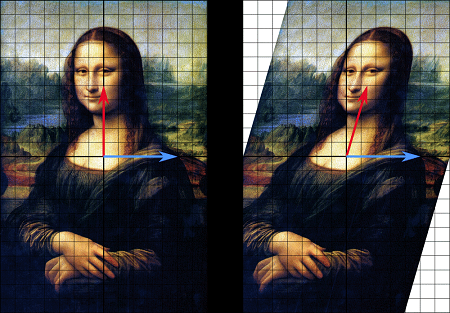Dyson discovered that the eigenvalue of these matrices are spaced apart in exactly the same manner as Montgomery conjecture of the nontrivial zeros of the zeta function. Means it also depends on Riemann hypotesis which is still in a major issue. Similar case left science today many unsolved problems that associated with.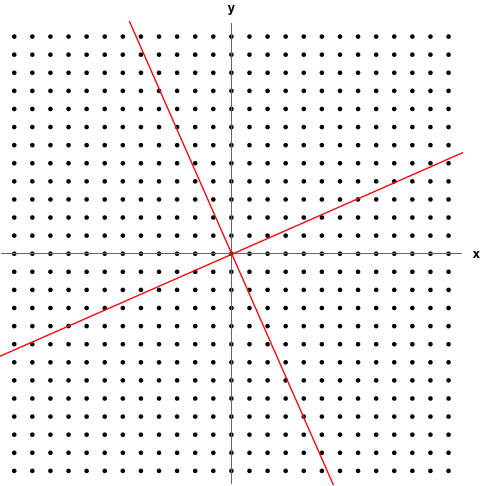The rank cannot be used to prove the theorem combinatorially, Dayson wrote an arithmetical coefficient similar to, but more recondite than, the rank of a partition; and called this hypothetical coefficient as the "crank" of the partition.

The values p(1),,,,,,p(8)} of the partition function (1, 2, 3, 5, 7, 11, 15, and 22) can be determined by counting the Young diagrams for the partitions of the numbers from 1 to 8 (Wikipedia).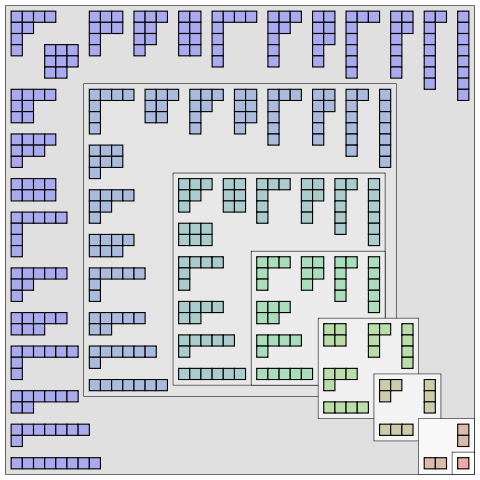Since 286 has the prime factors of 11 and 13 so it is also the product of the middle two numbers of True Prime Pairs. Therefore, the eigenvalue of the 11 parts also determines the equal size rotation of crank partitions of 13 prime identity.

``````\$True Prime Pairs:
(5,7), (11,13), (17,19)

layer | node | sub |  i  |  f
------+------+-----+----------
|      |     |  1  |     ◄-----------------------------
|      |  1  +-----+                                   |
|  1   |     |  2  | (5)                               |
|      |-----+-----+                                   |
|      |     |  3  |                                   |
1   +------+  2  +-----+----                               |
|      |     |  4  |                                   |
|      +-----+-----+                                   |
|  2   |     |  5  | (7)                               |
|      |  3  +-----+                                   |
|      |     |  6  |                                   |
------+------+-----+-----+------                             |
|      |     |  7  |                                   |
|      |  4  +-----+
|  3   |     |  8  | (11) ◄-- 11 parts of rank 10 = 110
|      +-----+-----+
|      |     |  9  |                                   |
2   +------|  5  +-----+-----                              |
|      |     |  10 |                                   |
|      |-----+-----+                                   |
|  4   |     |  11 | (13) ◄----------------------------
|      |  6  +-----+
|      |     |  12 |
------+------+-----+-----+-------------------- (36)
|      |     |  13 |
|      |  7  +-----+
|  5   |     |  14 | (17) ◄----------------------------
|      |-----+-----+                                   |
|      |     |  15 | 0 axis ◄-- partitions of the (13) ◄-- rotation
3   +------+  8  +-----+-----                              |
|      |     |  16 |      ◄----------------------------
|      |-----+-----+
|  6   |     |  17 | (19)
|      |  9  +-----+
|      |     |  18 |
------|------|-----+-----+-------------------- (72)
``````

Unfortunately the rotation of this eigenvalues deals with four-dimensional space-time which was already a big issue. Speculation is that the unfinished book of Ramanujan's partition, series of Dyson's solutions and hugh of Einstein's papers tend to solve it.

On the other hand, one does not yet have a mathematically complete example of a quantum gauge theory in 4D Space vs Time, nor even a precise definition of quantum gauge theory in four dimensions. Will this change in the 21st century? We hope so! (Clay Institute's - Official problem description).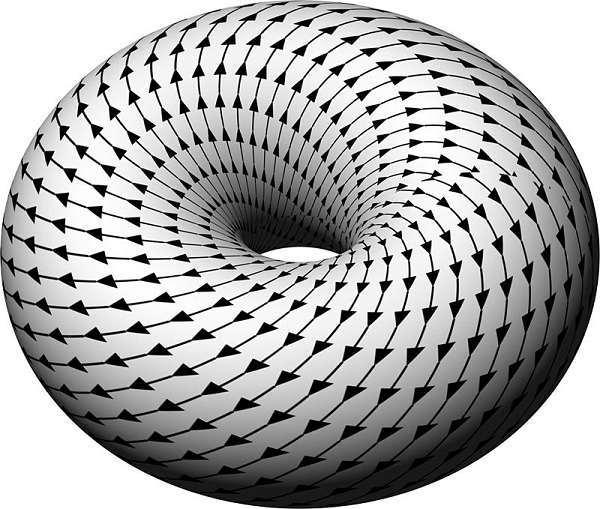More generally, the central problem is to determine when an equation in n-dimensional space has solutions. However at this point, we finaly found that the prime distribution has something to do with the subclasses of rank and crank partitions.

## The subclasses of each partitions

Dayson introduced the idea of rank of a partition to accomplish the task he set for himself. He made the following conjectures which were proved in 1954 by Peter Swinnerton-Dyer an English mathematician specialising in number theory.

Dayson's friend the neurologist and author Oliver Sacks said: "A favourite word of Freeman's about doing science and being creative is the word subversive (tending or intending to subvert or overthrow, destroy, or undermine an established or existing system, especially a legally constituted or a set of beliefs), and he's done that all his life (Wikipedia).

``````N(0, 5, 5n + 4) = N(1, 5, 5n + 4) = N(2, 5, 5n + 4) = N(3, 5, 5n + 4) = N(4, 5, 5n + 4)
N(0, 7, 7n + 5) = N(1, 7, 7n + 5) = N(2, 7, 7n + 5) = . . . = N(6, 7, 7n + 5)
``````

The concepts of rank and crank can both be used to classify partitions of certain integers into subclasses of equal size. The two concepts produce different subclasses of partitions. This is illustrated in the following two tables.

Although not in the form that Dayson have defined, it was found that the last problem on which Ramanujan worked on before his death was cranks. Berndt and his coauthors have given substantial evidence that Ramanujan knew about the function (Wikipedia).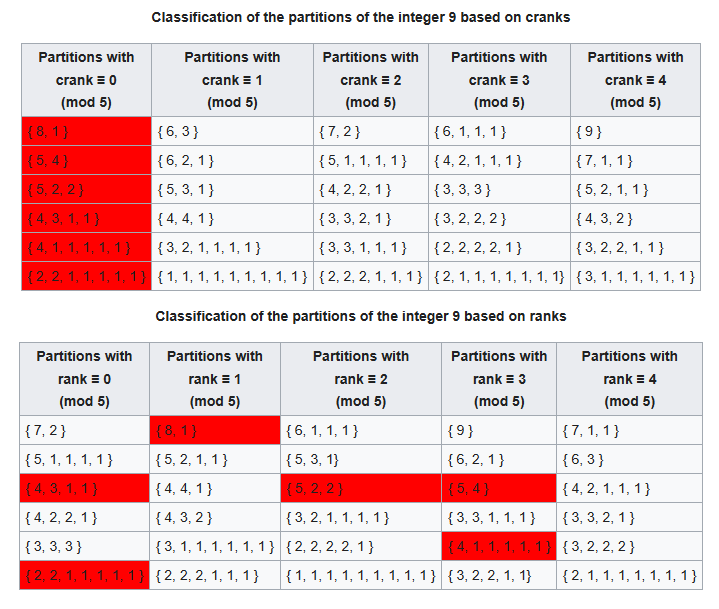The subclasses of partitions develops characters similar to the distribution of prime numbers. This results in a fundamental causal relation to the primes, systemically the products are entered into the position system.

The approach taken is to think of the solutions of an equation as a geometric object. For example, an equation in two variables defines a curve in the plane. More generally, an equation, or system of equations, in two or more variables defines a curve, a surface or some other such object in n-dimensional space (Wikipedia).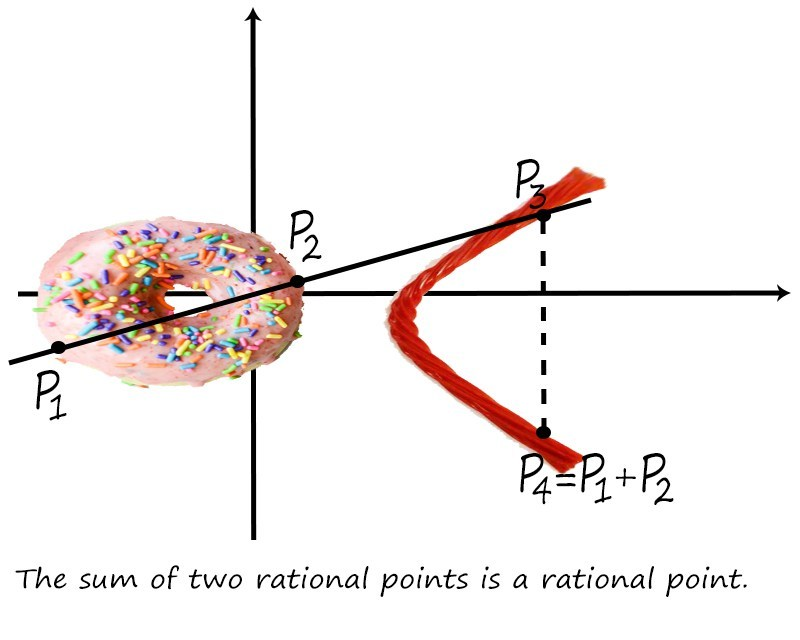Dyson has many series of formal solution. An explicitly time-dependent Schrödinger equation by iteration, and the time-ordering operator {T} as entity of basic importance of quantum mechanics, are also named after Dyson .

### Relation to the primes

The Ulam spiral or prime spiral is a graphical depiction of the set of prime numbers, devised by mathematician Stanisław Ulam in 1963 and popularized in Martin Gardner's Mathematical Games column in Scientific American a short time later.

Both Ulam and Gardner noted that the existence of such prominent lines is not unexpected, as lines in the spiral correspond to quadratic polynomials, and certain such polynomials, such as Euler's prime-generating polynomial x²-x+41, are believed to produce a high density of prime numbers. Nevertheless, the Ulam spiral is connected with major unsolved problems in number theory such as Landau's problems (Wikipedia).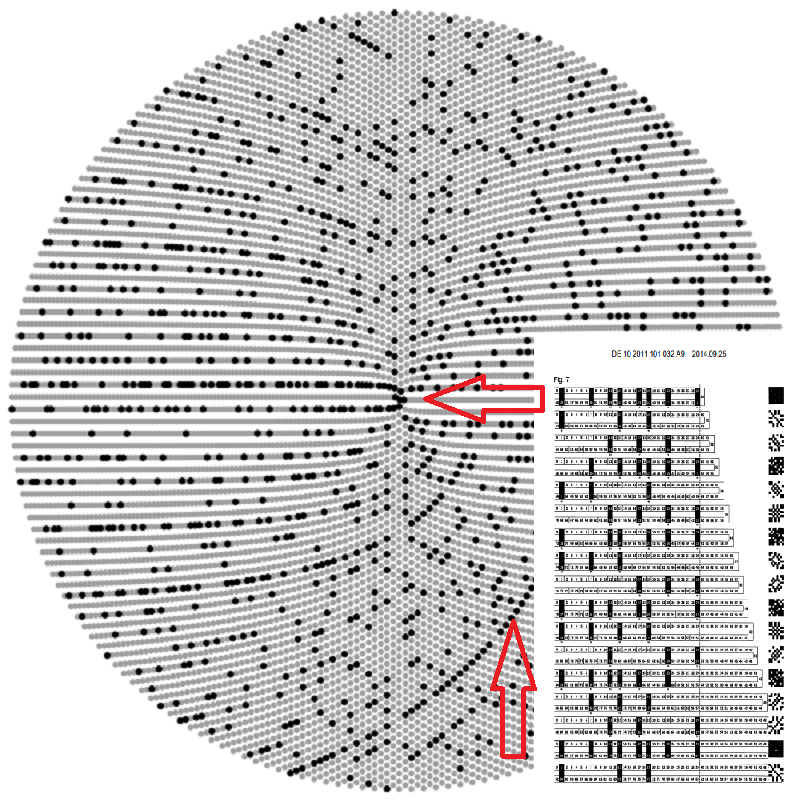According to the results of Princeton University USA in 1972, the distribution of the prime numbers shows in the Riemann zeta function between the position of its complex zeros and middle axis is identical with the rotation curve of energy distribution.

37 + 12 = 61 - 12 = 49 = 7 x 7 = d(13)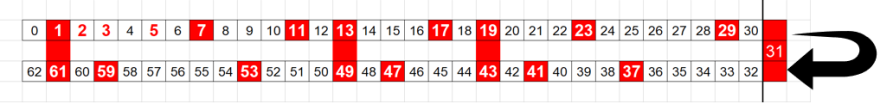In the second opposing member, the position 19 in the second term gives a redundant value of the template 7 of 7 × 7 = 49. The opposite prime position 11 as a prime number is now forced to determine a new axis-symmetrical zero position.

Note that when 77 contains ‘Lucky 7 and 11' as prime factors it is also the product of the middle two numbers of this sequence (11*7 = 77) (Prime Curios!).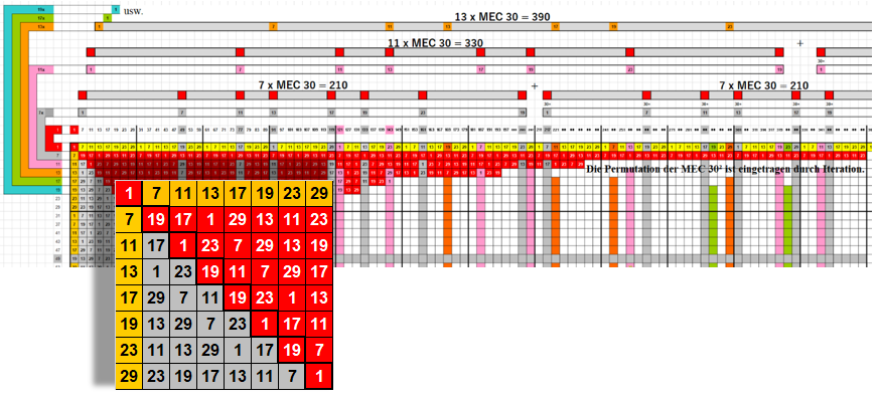When the subclasses of partitions are flatten out into a matrix, you want to take the Jacobian of each of a stack of targets with respect to a stack of sources, where the Jacobians for each target-source pair are independent .

It's possible to build a Hessian matrix for a Newton's method step using the Jacobian method. You would first flatten out its axes into a matrix, and flatten out the gradient into a vector (Tensorflow).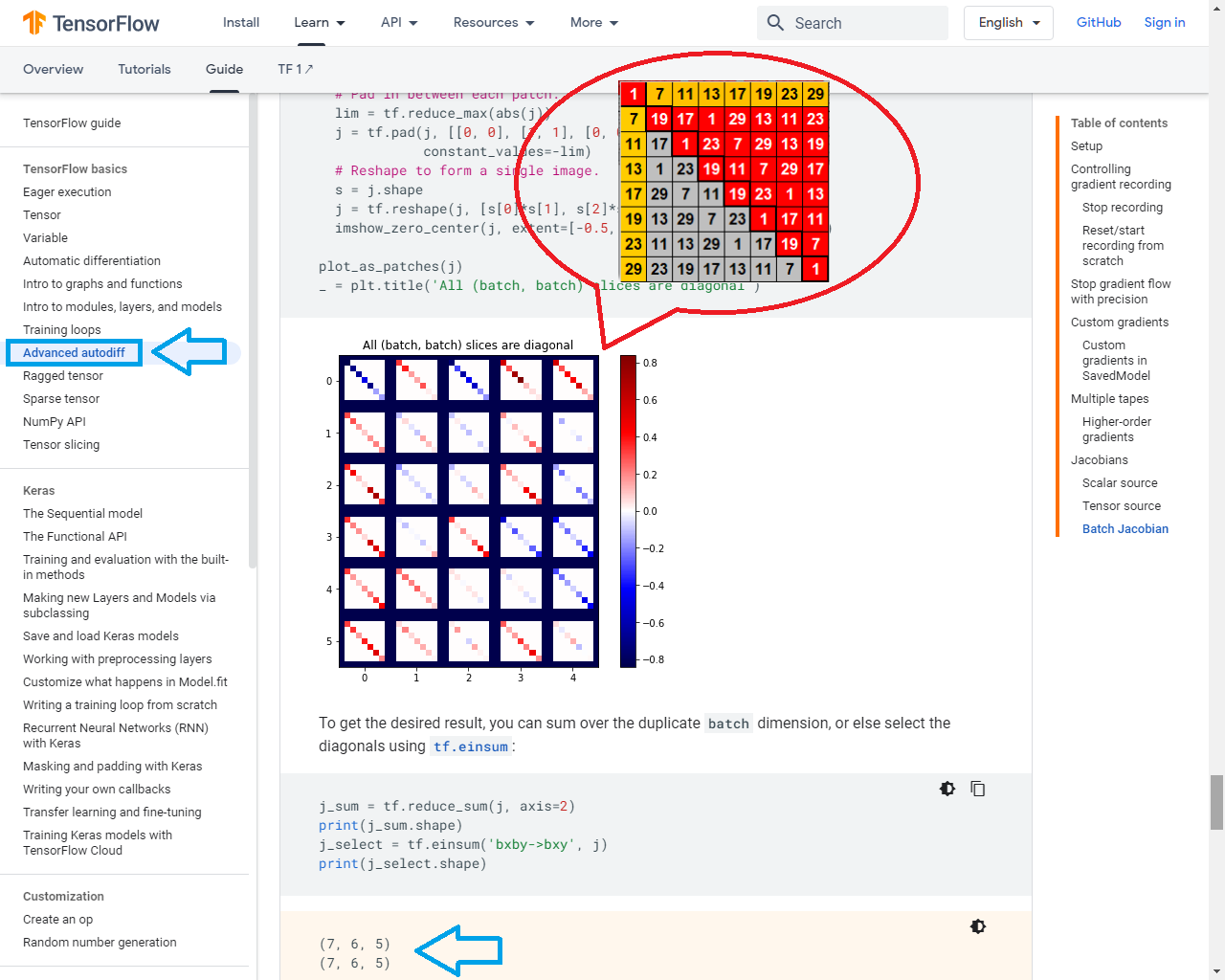This idea was done as the earliest in 1960s by Swinnerton-Dyer using the University of Cambridge Computer Laboratory to get the number of points modulo p (denoted by Np) for a large number of primes p on elliptic curves whose rank was known.

From these numerical results the conjecture predicts that the data should form a line of slope equal to the rank of the curve, which is 1 in this case drawn in red in red on the graph (Wikipedia).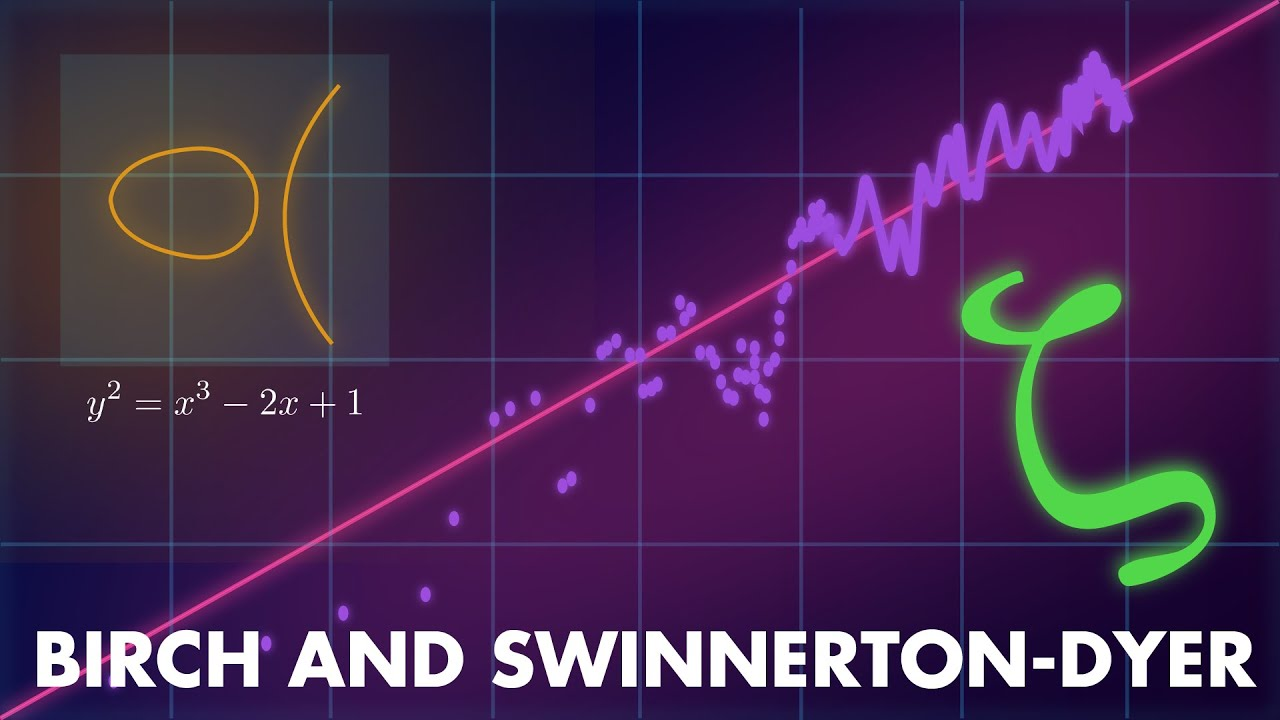It is stated that Np for a curve E with rank r obeys an asymptotic law and is still remain unsolved. Thus it would mean that using Euler's identity to get a definite pattern of prime distribution is still a long way to go.